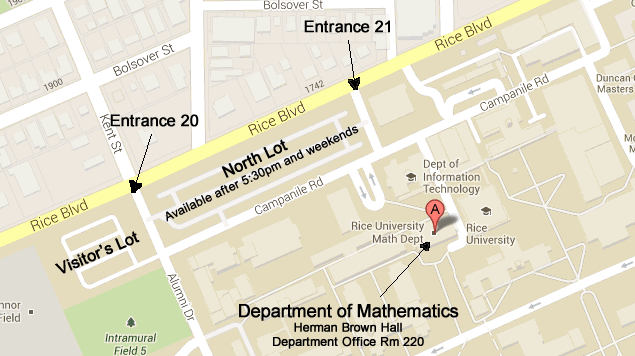# Which Upper Level Courses Should I Take?

#### Linear Algebra

Linear algebra underlies almost every other topic within mathematics, and is needed in virtually every field that uses mathematics. Potential math majors and minors should take Linear Algebra (Math 354 or Math 355) as early as possible after the initial calculus courses. (Note that Math 211 only covers a small amount of linear algebra and should not be viewed as sufficient.) Math 355 involves some proofs but also has a large computational component, while Math 354 is entirely proof-based, and is strongly recommended for anyone thinking about graduate study in mathematics. Math 354 is usually only offered in the fall semester, while Math 355 is offered in both semesters.

#### Proof-writing

The formal language of mathematics is one of mathematical proofs. Ideally, every student who studies mathematics should take a course which prepares them to read and write proofs. There are several courses which specifically aim to introduce students to proofs; among them are Math 221/222, Math 302, and Math 354. (Other courses may also provide this benefit, although this varies from year to year.)

#### Analysis and Algebra

Real analysis is the study of functions on the real line. It closely examines the properties of the real line and gives rigorous justifications for all of the results that one takes for granted when learning calculus. Abstract Algebra studies abstract algebraic structures such as groups, rings, and fields, which model algebraic operations such as addition and multiplication. Both subjects are at the core of higher mathematics and are strongly encouraged for all students.

Introductory analysis courses are Math 302, Math 321, and Math 331. Math 331 is an honors version of Math 321. Students who already have familiarity with proofs are able to skip Math 302 and enroll in Math 321 or 331.

The introductory algebra course is Math 356; it is offered in the fall. Math 357 (Algebra II) is a continuation of Math 356 in the spring semester, but one can take Math 356 without committing to taking Math 357.

#### Other Electives

There are many other 300- and 400-level electives offered. Some of them are advanced and should only be taken after one has had courses that provide preparation for writing proofs. Some involve almost no proof-writing whatsoever, and can often be taken by students who just have Math 211 and/or Math 212 as preparation. Some fall in the middle, and might vary from year to year.

The following table is a rough categorization of some of the electives offered, although students should check with the individual instructors to be sure about what level each course will be offered at. See the course catalog for descriptions of these courses.

Useful courses for students interested in math education or economics/finance

One Possible Degree Path for a B.A.

One Possible Degree Path for a B.S.

#### Some Mathematics Electives

Few/No Proofs Involved
Fall Spring
Math 381 (Intro to PDEs) Math 382 (Computational Complex Analysis)
Some Proofs Involved (can vary)
Fall Spring
Math 368 (Combinatorics) Math 365 (Number Theory)
Math 366 (Geometry)
Math 304 (Knot Theory)
Fall Spring
Math 371 (Lie Theory)
Math 374 (Representation Theory)
Math 401 (Curves and Surfaces)
Math 423 (PDEs)
Math 425 (Integration Theory)
Math 370 (Calculus on Manifolds)
Math 373 (Elliptic Curves)
Math 427 (Complex Analysis)
Math 443 (Topology)Large Map

Tel (713) 348-4829